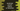# JavaScript program to convert Celsius to Fahrenheit## JavaScript program to convert Celsius to Fahrenheit:

In this post, we will learn how to convert a celsius value to fahrenheit. We will also use HTML, CSS to create a HTML page that can be opened in the browser and do the conversion by taking user inputs.

For converting celsius to fahrenheit, we will take the value of celsius as input from the user and do the conversion.

### Formula to convert celsius to fahrenheit:

Below formula we can use to convert a celsius value to fahrenheit:

``fahrenheit = (1.8 * celsius) + 32``

### JavaScript program:

Below JavaScript program shows how we can convert a celsius to fahrenheit value:

``````const celsius = 100;

const fahrenheit = 1.8 * celsius + 32;

console.log(`\${celsius} Celsius = \${fahrenheit} Fahrenheit`);``````

If you run this program, it will print:

``100 Celsius = 212 Fahrenheit``

### Using HTML, CSS and JavaScript:

We can also add this JavaScript code in a HTML file and read the celsius value as input from the user. Create one new file index.html and add the below content:

``````<!DOCTYPE html>
<html lang="en">
<script>
function convertToFahrenheit() {
var fahRenheit = 1.8 * document.getElementById("input").value + 32;

document.getElementById("result").innerHTML = fahRenheit;
}
</script>